# NCERT Solutions (Ex - 4.4, 4.5) - Practical Geometry, Maths, Class 8 Notes - Class 8

## Class 8: NCERT Solutions (Ex - 4.4, 4.5) - Practical Geometry, Maths, Class 8 Notes - Class 8

The document NCERT Solutions (Ex - 4.4, 4.5) - Practical Geometry, Maths, Class 8 Notes - Class 8 is a part of Class 8 category.
All you need of Class 8 at this link: Class 8

Exercise 4.4

Question 1: Construct the following quadrilaterals:

DE = 4 cm, EA = 5 cm, AR = 4.5 cm, ∠E = 60o ,∠A = 90o

TR = 3.5 cm, RU = 3 cm, UE = 4 cm, ∠ R= 75°, ∠ U = 120°

(i) Given: DE = 4cm, EA = 5cm, AR = 4.5 cm,  ∠E = 60% ,∠A = 90o

To construct:

A quadrilateral DEAR. Steps of construction:

(a) Draw a line segment DE = 4 cm.

(b) At point E, construct an angle of 60o.

(c) Taking radius 5 cm, draw an arc from point E which intersects at A.

(d) Construct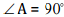, draw an arc of radius 4.5 cm with centre A which intersect at R.

(e) Join RD.

It is the required quadrilateral DEAR.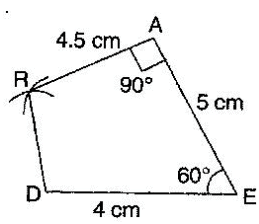(ii) Given: TR = 3.5 cm, RU = 3 cm, UE = 4 cm, ∠R = 75°, ∠U = 120o

Steps of construction:

1. Draw a line segment TR = 3.5 cm.
2. Construct an angle 75o at R and draw an arc of radius 3 cm with R as centre, which intersects at U.
3. Construct an angle of 120° at U and produce the side UE.
4. Draw an arc of radius 4 cm with U as centre.
5. Join UE and TE.

It is the required quadrilateral TRUE.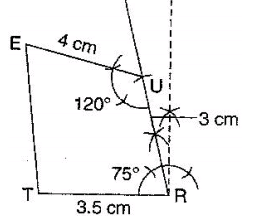Exercise 4.5

Question 1:

Draw the following: The square READ with RE = 5.1 cm.

Given: RE = 5.1 cm.

Steps of construction:

(i) Draw RE = 5.1 cm.

(ii) At point E, construct an angle of 90o and draw an arc of radius 5.1 cm, which intersects at point A.

(iii) At point R, draw an arc of radius 5.1 cm at point A, draw another arc of radius 5.1 cm which intersects the first arc at point D.

It is the required square READ,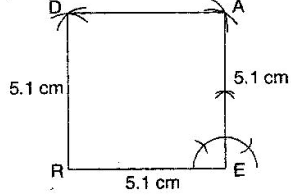Question 2:

Draw the following: A rhombus whose diagonals are 5.2 cm and 6.4 cm.

Given: Diagonals of a rhombus AC = 5.2 cm and BD = 6.4 cm.

To construct: A rhombus ABCD.

Steps of construction:

(a) Draw AC = 5.2 cm and draw perpendicular bisectors on AC.

(b) Since, diagonals bisect at mid-point O, therefore get half of 6.4 cm, i.e., 3.2 cm.

(c) Draw two arcs on both sides of AC of radius 3.2 cm from intersection point O, which intersects at B and D.

(d) Join AB, BC, CD and DA.

It is required rhombus ABCD.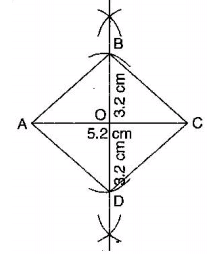Question 3:

Draw the following: A rectangle with adjacent sides of length 5 cm and 4 cm.

Given: MN = 5 cm and MP = 4 cm.

To construct: A rectangle MNOP

Steps of construction:

(a) Draw a segment MN = 5 cm.

(b) At points M and N, draw perpendiculars of lengths 4 cm and produce them.

(c) Taking centres M and N, draw two arcs of 4 cm each, which intersect P and Q respectively.

(d) Join side PO.

It is required rectangle MNOP.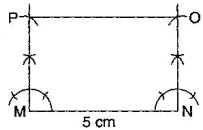Question 4:

Draw the following: A parallelogram OKAY where OK = 5.5 cm and KA = 4.2 cm.

Given: OK = 5.5 cm and KA = 4.2 cm.

To construct: A parallelogram OKAY.

Steps of construction:

(a) Draw a line segment OK = 5.5 cm.

(b) Draw an angle of 90 at K and draw an arc of radius KA = 4.2 cm, which intersects at point A.

(c) Draw another arc of radius AY = 5.5 cm and at point O, draw another arc of radius 4.2 cm which intersect at Y.

(d) Join AY and OY.

It is the required parallelogram OKAY.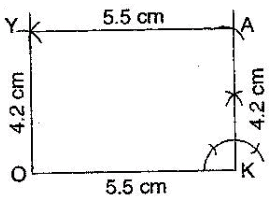The document NCERT Solutions (Ex - 4.4, 4.5) - Practical Geometry, Maths, Class 8 Notes - Class 8 is a part of Class 8 category.
All you need of Class 8 at this link: Class 8Use Code STAYHOME200 and get INR 200 additional OFF

Track your progress, build streaks, highlight & save important lessons and more!

,

,

,

,

,

,

,

,

,

,

,

,

,

,

,

,

,

,

,

,

,

,

,

,

,

,

,

,

,

,

;